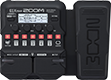# Tuncowsky's Tremolo

Discussion in 'Zoom G1/G1X Four' started by tuncowsky, Jan 16, 2022.

1. Tuncowsky's TremoloDevice: Zoom G1X Four
Firmware: 2.00

Name on device: TremTunco
Optimized for: Phones/Speaker

Effects chain:tuncowsky tremolo

Effect: "Tremolo" (Modulation), active - "yes"
"Wave" = TUBE
"Depth" = 90
"Rate" = 50
"Volume" = 98

Effect: "SoftEcho" (Delay), active - "yes"
"MOD" = On
"Time" = 406
"F.B." = 47
"Mix" = 79

Effect: "OptComp" (Dynamics), active - "yes"
"Drive" = 7
"Lo" = 38
"Hi" = 50
"Volume" = 69

Effect: "Gt GEQ 7" (Filter), active - "yes"
"100" = -4.0
"200" = -2.5
"400" = 3.0
"800" = -2.5
"1.6k" = -1.0
"3.2k" = 2.5
"6.4k" = 1.0
"VOL" = 80

Patch Volume: 77